# ppt.pptx

26 de Mar de 2023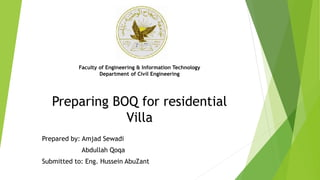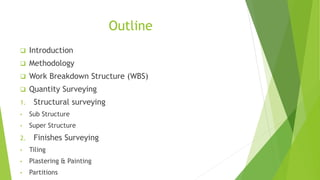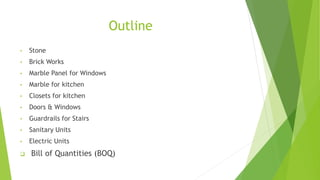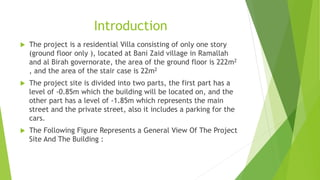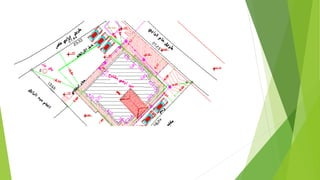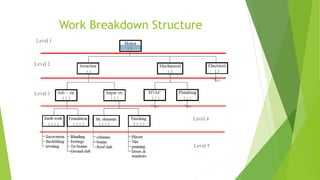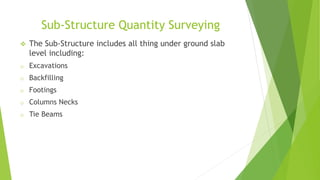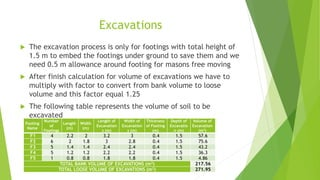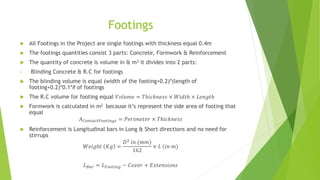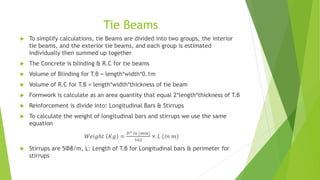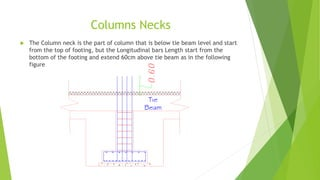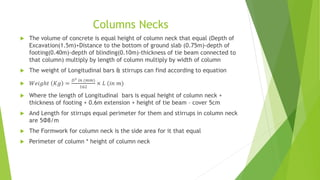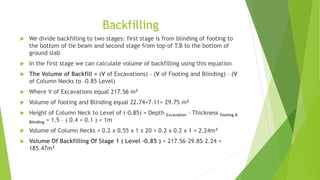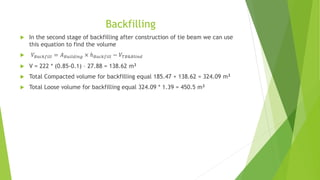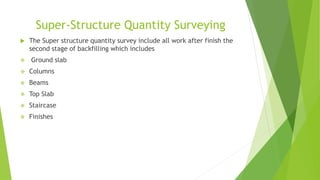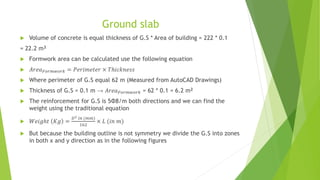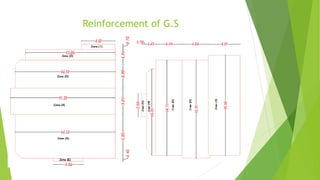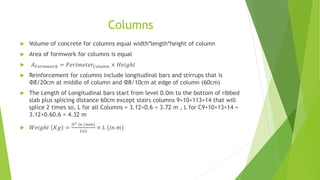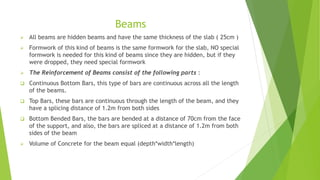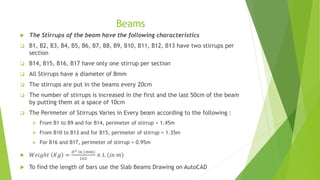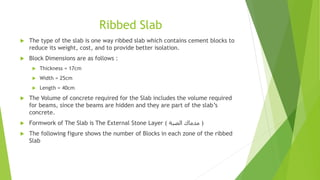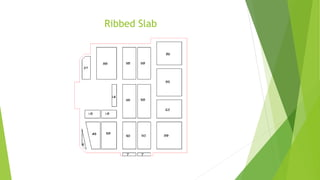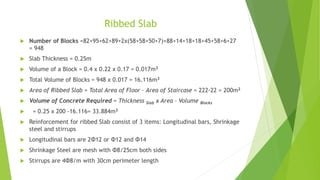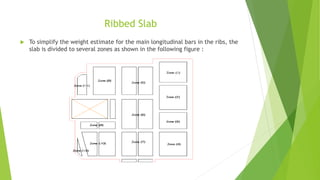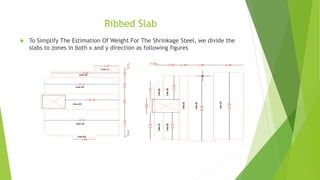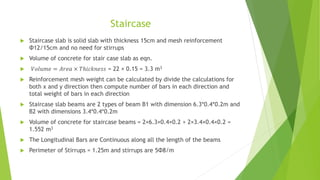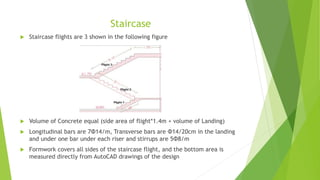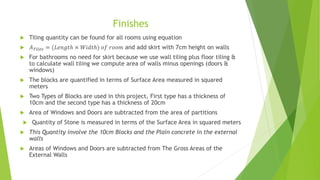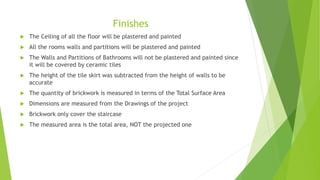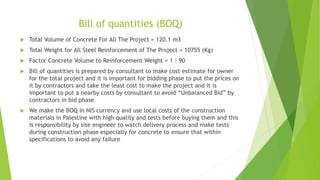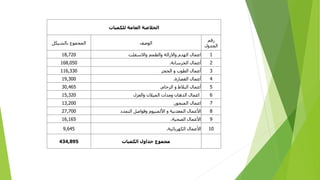1 de 32

### ppt.pptx

1. Faculty of Engineering & Information Technology Department of Civil Engineering Preparing BOQ for residential Villa Prepared by: Amjad Sewadi Abdullah Qoqa Submitted to: Eng. Hussein AbuZant
2. Outline  Introduction  Methodology  Work Breakdown Structure (WBS)  Quantity Surveying 1. Structural surveying • Sub Structure • Super Structure 2. Finishes Surveying • Tiling • Plastering & Painting • Partitions
3. Outline • Stone • Brick Works • Marble Panel for Windows • Marble for kitchen • Closets for kitchen • Doors & Windows • Guardrails for Stairs • Sanitary Units • Electric Units  Bill of Quantities (BOQ)
4. Introduction  The project is a residential Villa consisting of only one story (ground floor only ), located at Bani Zaid village in Ramallah and al Birah governorate, the area of the ground floor is 222m2 , and the area of the stair case is 22m2  The project site is divided into two parts, the first part has a level of -0.85m which the building will be located on, and the other part has a level of -1.85m which represents the main street and the private street, also it includes a parking for the cars.  The Following Figure Represents a General View Of The Project Site And The Building :
5. Methodology Project Break Down Structure into Small Work Packages Summarizng Relevant Information for Each Work Package Determining the Method of Estimation of Quantities for Each Work Package Finding the Cost Considering the Common Cost in The Palestinian Market of Construction Works Summing Up Information to Find The Total Quantites and Costs
6. Work Breakdown Structure
7. Sub-Structure Quantity Surveying  The Sub-Structure includes all thing under ground slab level including: o Excavations o Backfilling o Footings o Columns Necks o Tie Beams
8. Excavations  The excavation process is only for footings with total height of 1.5 m to embed the footings under ground to save them and we need 0.5 m allowance around footing for masons free moving  After finish calculation for volume of excavations we have to multiply with factor to convert from bank volume to loose volume and this factor equal 1.25  The following table represents the volume of soil to be excavated Footing Name Number of Footings Length (m) Width (m) Length of Excavation s (m) Width of Excavation s (m) Thickness of Footing (m) Depth of Excavatio n (m) Volume of Excavation (m3) F1 4 2.2 2 3.2 3 0.4 1.5 57.6 F2 6 2 1.8 3 2.8 0.4 1.5 75.6 F3 5 1.4 1.4 2.4 2.4 0.4 1.5 43.2 F4 5 1.2 1.2 2.2 2.2 0.4 1.5 36.3 F5 1 0.8 0.8 1.8 1.8 0.4 1.5 4.86 TOTAL BANK VOLUME OF EXCAVATIONS (m3) 217.56 TOTAL LOOSE VOLUME OF EXCAVATIONS (m3) 271.95
9. Footings  All Footings in the Project are single footings with thickness equal 0.4m  The footings quantities consist 3 parts: Concrete, Formwork & Reinforcement  The quantity of concrete is volume in & m3 it divides into 2 parts: • Blinding Concrete & R.C for footings  The blinding volume is equal (width of the footing+0.2)*(length of footing+0.2)*0.1*# of footings  The R.C volume for footing equal 𝑉𝑜𝑙𝑢𝑚𝑒 = 𝑇ℎ𝑖𝑐𝑘𝑛𝑒𝑠𝑠 × 𝑊𝑖𝑑𝑡ℎ × 𝐿𝑒𝑛𝑔𝑡ℎ  Formwork is calculated in m2 because it’s represent the side area of footing that equal 𝐴𝐶𝑜𝑛𝑡𝑎𝑐𝑡𝐹𝑜𝑜𝑡𝑖𝑛𝑔𝑠 = 𝑃𝑒𝑟𝑖𝑚𝑒𝑡𝑒𝑟 × 𝑇ℎ𝑖𝑐𝑘𝑛𝑒𝑠𝑠  Reinforcement is Longitudinal bars in Long & Short directions and no need for stirrups 𝑊𝑒𝑖𝑔ℎ𝑡 𝐾𝑔 = 𝐷2 𝑖𝑛 (𝑚𝑚) 162 × 𝐿 (𝑖𝑛 𝑚) 𝐿𝐵𝑎𝑟 = 𝐿𝐹𝑜𝑜𝑡𝑖𝑛𝑔 − 𝐶𝑜𝑣𝑒𝑟 + 𝐸𝑥𝑡𝑒𝑛𝑠𝑖𝑜𝑛𝑠
10. Tie Beams  To simplify calculations, tie Beams are divided into two groups, the interior tie beams, and the exterior tie beams, and each group is estimated individually then summed up together  The Concrete is blinding & R.C for tie beams  Volume of Blinding for T.B = length*width*0.1m  Volume of R.C for T.B = length*width*thickness of tie beam  Formwork is calculate as an area quantity that equal 2*length*thickness of T.B  Reinforcement is divide into: Longitudinal Bars & Stirrups  To calculate the weight of longitudinal bars and stirrups we use the same equation 𝑊𝑒𝑖𝑔ℎ𝑡 𝐾𝑔 = 𝐷2 𝑖𝑛 (𝑚𝑚) 162 × 𝐿 (𝑖𝑛 𝑚)  Stirrups are 5Φ8/m, L: Length of T.B for Longitudinal bars & perimeter for stirrups
11. Columns Necks  The Column neck is the part of column that is below tie beam level and start from the top of footing, but the Longitudinal bars Length start from the bottom of the footing and extend 60cm above tie beam as in the following figure
12. Columns Necks  The volume of concrete is equal height of column neck that equal (Depth of Excavation(1.5m)+Distance to the bottom of ground slab (0.75m)-depth of footing(0.40m)-depth of blinding(0.10m)-thickness of tie beam connected to that column) multiply by length of column multiply by width of column  The weight of Longitudinal bars & stirrups can find according to equation  𝑊𝑒𝑖𝑔ℎ𝑡 𝐾𝑔 = 𝐷2 𝑖𝑛 (𝑚𝑚) 162 × 𝐿 (𝑖𝑛 𝑚)  Where the length of Longitudinal bars is equal height of column neck + thickness of footing + 0.6m extension + height of tie beam – cover 5cm  And Length for stirrups equal perimeter for them and stirrups in column neck are 5Φ8/m  The Formwork for column neck is the side area for it that equal  Perimeter of column * height of column neck
13. Backfilling  We divide backfilling to two stages: first stage is from blinding of footing to the bottom of tie beam and second stage from top of T.B to the bottom of ground slab  In the first stage we can calculate volume of backfilling using this equation  The Volume of Backfill = (V of Excavations) – (V of Footing and Blinding) – (V of Column Necks to -0.85 Level)  Where V of Excavations equal 217.56 m3  Volume of footing and Blinding equal 22.74+7.11= 29.75 m2  Height of Column Neck to Level of (-0.85) = Depth Excavation – Thickness Footing & Blinding = 1.5 – ( 0.4 + 0.1 ) = 1m  Volume of Column Necks = 0.2 x 0.55 x 1 x 20 + 0.2 x 0.2 x 1 = 2.24m3  Volume Of Backfilling Of Stage 1 ( Level -0.85 ) = 217.56–29.85–2.24 = 185.47m3
14. Backfilling  In the second stage of backfilling after construction of tie beam we can use this equation to find the volume  𝑉𝐵𝑎𝑐𝑘𝑓𝑖𝑙𝑙 = 𝐴𝐵𝑢𝑖𝑙𝑑𝑖𝑛𝑔 × ℎ𝐵𝑎𝑐𝑘𝑓𝑖𝑙𝑙 − 𝑉𝑇𝐵&𝐵𝑙𝑖𝑛𝑑  V = 222 * (0.85-0.1) – 27.88 = 138.62 m3  Total Compacted volume for backfilling equal 185.47 + 138.62 = 324.09 m3  Total Loose volume for backfilling equal 324.09 * 1.39 = 450.5 m3
15. Super-Structure Quantity Surveying  The Super structure quantity survey include all work after finish the second stage of backfilling which includes  Ground slab  Columns  Beams  Top Slab  Staircase  Finishes
16. Ground slab  Volume of concrete is equal thickness of G.S * Area of building = 222 * 0.1 = 22.2 m3  Formwork area can be calculated use the following equation  𝐴𝑟𝑒𝑎𝐹𝑜𝑟𝑚𝑤𝑜𝑟𝑘 = 𝑃𝑒𝑟𝑖𝑚𝑒𝑡𝑒𝑟 × 𝑇ℎ𝑖𝑐𝑘𝑛𝑒𝑠𝑠  Where perimeter of G.S equal 62 m (Measured from AutoCAD Drawings)  Thickness of G.S = 0.1 m → 𝐴𝑟𝑒𝑎𝐹𝑜𝑟𝑚𝑤𝑜𝑟𝑘 = 62 * 0.1 = 6.2 m2  The reinforcement for G.S is 5Φ8/m both directions and we can find the weight using the traditional equation  𝑊𝑒𝑖𝑔ℎ𝑡 𝐾𝑔 = 𝐷2 𝑖𝑛 (𝑚𝑚) 162 × 𝐿 (𝑖𝑛 𝑚)  But because the building outline is not symmetry we divide the G.S into zones in both x and y direction as in the following figures
17. Reinforcement of G.S
18. Columns  Volume of concrete for columns equal width*length*height of column  Area of formwork for columns is equal  𝐴𝐹𝑜𝑟𝑚𝑤𝑜𝑟𝑘 = 𝑃𝑒𝑟𝑖𝑚𝑒𝑡𝑒𝑟𝐶𝑜𝑙𝑢𝑚𝑛 × 𝐻𝑒𝑖𝑔ℎ𝑡  Reinforcement for columns include longitudinal bars and stirrups that is Φ8/20cm at middle of column and Φ8/10cm at edge of column (60cm)  The Length of Longitudinal bars start from level 0.0m to the bottom of ribbed slab plus splicing distance 60cm except stairs columns 9+10+113+14 that will splice 2 times so, L for all Columns = 3.12+0.6 = 3.72 m , L for C9+10+13+14 = 3.12+0.60.6 = 4.32 m  𝑊𝑒𝑖𝑔ℎ𝑡 𝐾𝑔 = 𝐷2 𝑖𝑛 (𝑚𝑚) 162 × 𝐿 (𝑖𝑛 𝑚)
19. Beams  All beams are hidden beams and have the same thickness of the slab ( 25cm )  Formwork of this kind of beams is the same formwork for the slab, NO special formwork is needed for this kind of beams since they are hidden, but if they were dropped, they need special formwork  The Reinforcement of Beams consist of the following parts :  Continuous Bottom Bars, this type of bars are continuous across all the length of the beams.  Top Bars, these bars are continuous through the length of the beam, and they have a splicing distance of 1.2m from both sides  Bottom Bended Bars, the bars are bended at a distance of 70cm from the face of the support, and also, the bars are spliced at a distance of 1.2m from both sides of the beam  Volume of Concrete for the beam equal (depth*width*length)
20. Beams  The Stirrups of the beam have the following characteristics  B1, B2, B3, B4, B5, B6, B7, B8, B9, B10, B11, B12, B13 have two stirrups per section  B14, B15, B16, B17 have only one stirrup per section  All Stirrups have a diameter of 8mm  The stirrups are put in the beams every 20cm  The number of stirrups is increased in the first and the last 50cm of the beam by putting them at a space of 10cm  The Perimeter of Stirrups Varies in Every beam according to the following :  From B1 to B9 and for B14, perimeter of stirrup = 1.45m  From B10 to B13 and for B15, perimeter of stirrup = 1.35m  For B16 and B17, perimeter of stirrup = 0.95m  𝑊𝑒𝑖𝑔ℎ𝑡 𝐾𝑔 = 𝐷2 𝑖𝑛 (𝑚𝑚) 162 × 𝐿 (𝑖𝑛 𝑚)  To find the length of bars use the Slab Beams Drawing on AutoCAD
21. Ribbed Slab  The type of the slab is one way ribbed slab which contains cement blocks to reduce its weight, cost, and to provide better isolation.  Block Dimensions are as follows :  Thickness = 17cm  Width = 25cm  Length = 40cm  The Volume of concrete required for the Slab includes the volume required for beams, since the beams are hidden and they are part of the slab’s concrete.  Formwork of The Slab is The External Stone Layer ( ‫الصبة‬ ‫مدماك‬ )  The following figure shows the number of Blocks in each zone of the ribbed Slab
22. Ribbed Slab
23. Ribbed Slab  Number of Blocks =82+95+62+89+2x(58+58+50+7)+88+14+18+18+45+58+6+27 = 948  Slab Thickness = 0.25m  Volume of a Block = 0.4 x 0.22 x 0.17 = 0.017m3  Total Volume of Blocks = 948 x 0.017 = 16.116m3  Area of Ribbed Slab = Total Area of Floor – Area of Staircase = 222-22 = 200m2  Volume of Concrete Required = Thickness Slab x Area – Volume Blocks  = 0.25 x 200 -16.116= 33.884m3  Reinforcement for ribbed Slab consist of 3 items: Longitudinal bars, Shrinkage steel and stirrups  Longitudinal bars are 2Φ12 or Φ12 and Φ14  Shrinkage Steel are mesh with Φ8/25cm both sides  Stirrups are 4Φ8/m with 30cm perimeter length
24. Ribbed Slab  To simplify the weight estimate for the main longitudinal bars in the ribs, the slab is divided to several zones as shown in the following figure :
25. Ribbed Slab  To Simplify The Estimation Of Weight For The Shrinkage Steel, we divide the slabs to zones in both x and y direction as following figures
26. Staircase  Staircase slab is solid slab with thickness 15cm and mesh reinforcement Φ12/15cm and no need for stirrups  Volume of concrete for stair case slab as eqn.  𝑉𝑜𝑙𝑢𝑚𝑒 = 𝐴𝑟𝑒𝑎 × 𝑇ℎ𝑖𝑐𝑘𝑛𝑒𝑠𝑠 = 22 × 0.15 = 3.3 m3  Reinforcement mesh weight can be calculated by divide the calculations for both x and y direction then compute number of bars in each direction and total weight of bars in each direction  Staircase slab beams are 2 types of beam B1 with dimension 6.3*0.4*0.2m and B2 with dimensions 3.4*0.4*0.2m  Volume of concrete for staircase beams = 2×6.3×0.4×0.2 + 2×3.4×0.4×0.2 = 1.552 m3  The Longitudinal Bars are Continuous along all the length of the beams  Perimeter of Stirrups = 1.25m and stirrups are 5Φ8/m
27. Staircase  Staircase flights are 3 shown in the following figure  Volume of Concrete equal (side area of flight*1.4m + volume of Landing)  Longitudinal bars are 7Φ14/m, Transverse bars are Φ14/20cm in the landing and under one bar under each riser and stirrups are 5Φ8/m  Formwork covers all sides of the staircase flight, and the bottom area is measured directly from AutoCAD drawings of the design
28. Finishes  Tiling quantity can be found for all rooms using equation  𝐴𝑇𝑖𝑙𝑒𝑠 = (𝐿𝑒𝑛𝑔𝑡ℎ × 𝑊𝑖𝑑𝑡ℎ) 𝑜𝑓 𝑟𝑜𝑜𝑚 and add skirt with 7cm height on walls  For bathrooms no need for skirt because we use wall tiling plus floor tiling & to calculate wall tiling we compute area of walls minus openings (doors & windows)  The blocks are quantified in terms of Surface Area measured in squared meters  Two Types of Blocks are used in this project, First type has a thickness of 10cm and the second type has a thickness of 20cm  Area of Windows and Doors are subtracted from the area of partitions  Quantity of Stone is measured in terms of the Surface Area in squared meters  This Quantity involve the 10cm Blocks and the Plain concrete in the external walls  Areas of Windows and Doors are subtracted from The Gross Areas of the External Walls
29. Finishes  The Ceiling of all the floor will be plastered and painted  All the rooms walls and partitions will be plastered and painted  The Walls and Partitions of Bathrooms will not be plastered and painted since it will be covered by ceramic tiles  The height of the tile skirt was subtracted from the height of walls to be accurate  The quantity of brickwork is measured in terms of the Total Surface Area  Dimensions are measured from the Drawings of the project  Brickwork only cover the staircase  The measured area is the total area, NOT the projected one
30. Bill of quantities (BOQ)  Total Volume of Concrete For All The Project = 120.1 m3  Total Weight for All Steel Reinforcement of The Project = 10755 (Kg)  Factor Concrete Volume to Reinforcement Weight = 1 : 90  Bill of quantities is prepared by consultant to make cost estimate for owner for the total project and it is important for bidding phase to put the prices on it by contractors and take the least cost to make the project and it is important to put a nearby costs by consultant to avoid “Unbalanced Bid” by contractors in bid phase  We make the BOQ in NIS currency and use local costs of the construction materials in Palestine with high quality and tests before buying them and this is responsibility by site engineer to watch delivery process and make tests during construction phase especially for concrete to ensure that within specifications to avoid any failure
31. ‫للكميات‬ ‫العامة‬ ‫الخالصة‬ ‫رقم‬ ‫الجدول‬ ‫الوصف‬ ‫بالشيكل‬ ‫المجموع‬ 1 ‫واالسفلت‬ ‫والطمم‬ ‫واالزالة‬ ‫الهدم‬ ‫اعمال‬ 18,720 2 ‫الخرسانة‬ ‫أعمال‬ . 168,050 3 ‫أعمال‬ ‫الطوب‬ ‫الحجر‬ ‫و‬ 116,330 4 ‫القصارة‬ ‫أعمال‬ . 19,300 5 ‫الرخام‬ ‫و‬ ‫البالط‬ ‫أعمال‬ . 30,465 6 ‫والعزل‬ ‫الميالن‬ ‫ومدات‬ ‫الدهان‬ ‫اعمال‬ 15,320 7 ‫المنجور‬ ‫اعمال‬ . 13,200 8 ‫التمدد‬ ‫وفواصل‬ ‫األلمنيوم‬ ‫و‬ ‫المعدنية‬ ‫األعمال‬ 27,700 9 ‫الصحية‬ ‫األعمال‬ . 16,165 10 ‫الكهربائية‬ ‫األعمال‬ . 9,645 ‫الكميات‬ ‫جداول‬ ‫مجموع‬ 434,895